# Dependent Events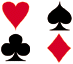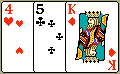Experiment 1: A card is chosen at random from a standard deck of 52 playing cards. Without replacing it, a second card is chosen. What is the probability that the first card chosen is a queen and the second card chosen is a jack?

Analysis: The probability that the first card is a queen is 4 out of 52. However, if the first card is not replaced, then the second card is chosen from only 51 cards. Accordingly, the probability that the second card is a jack given that the first card is a queen is 4 out of 51.

Conclusion: The outcome of choosing the first card has affected the outcome of choosing the second card, making these events dependent.

Definition: Two events are dependent if the outcome or occurrence of the first affects the outcome or occurrence of the second so that the probability is changed.

Now that we have accounted for the fact that there is no replacement, we can find the probability of the dependent events in Experiment 1 by multiplying the probabilities of each event.Experiment 1: A card is chosen at random from a standard deck of 52 playing cards. Without replacing it, a second card is chosen. What is the probability that the first card chosen is a queen and the second card chosen is a jack?

Probabilities:

 P(queen on first pick) = 4 52 P(jack on 2nd pick given queen on 1st pick) = 4 51 P(queen and jack) = 4 · 4 = 16 = 4 52 51 2652 663

Experiment 1 involved two compound, dependent events. The probability of choosing a jack on the second pick given that a queen was chosen on the first pick is called a conditional probability.

The conditional probability of an event B in relationship to an event A is the probability that event B occurs given that event A has already occurred. The notation for conditional probability is P(B|A) [pronounced as The probability of event B given A].

The notation used above does not mean that B is divided by A. It means the probability of event B given that event A has already occurred. To find the probability of the two dependent events, we use a modified version of Multiplication Rule 1, which was presented in the last lesson.

Multiplication Rule 2: When two events, A and B, are dependent, the probability of both occurring is:

P(A and B)  =  P(A) · P(B|A)

Let's look at some experiments in which we can apply this rule.Experiment 2: Mr. Parietti needs two students to help him with a science demonstration for his class of 18 girls and 12 boys. He randomly chooses one student who comes to the front of the room. He then chooses a second student from those still seated. What is the probability that both students chosen are girls?

Probabilities P(Girl 1 and Girl 2) = P(Girl 1) and P(Girl 2|Girl 1)

 = 18 · 17 30 29= 306 870= 51 145Experiment 3: In a shipment of 20 computers, 3 are defective. Three computers are randomly selected and tested. What is the probability that all three are defective if the first and second ones are not replaced after being tested?

Probabilities: P(3 defectives)  =

 3 · 2 · 1 = 6 = 1 20 19 18 6840 1140Experiment 4: Four cards are chosen at random from a deck of 52 cards without replacement. What is the probability of choosing a ten, a nine, an eight and a seven in order?

Probabilities: P(10 and 9 and 8 and 7)  =

 4 · 4 · 4 · 4 = 256 = 32 52 51 50 49 6,497,400 812,175Experiment 5: Three cards are chosen at random from a deck of 52 cards without replacement. What is the probability of choosing 3 aces?

Probabilities: P(3 aces)  =

 4 · 3 · 2 = 24 = 1 52 51 50 132,600 5,525

Summary: Two events are dependent if the outcome or occurrence of the first affects the outcome or occurrence of the second so that the probability is changed. The conditional probability of an event B in relationship to an event A is the probability that event B occurs given that event A has already occurred. The notation for conditional probability is P(B|A). When two events, A and B, are dependent, the probability of both occurring is: P(A and B)  =  P(A) · P(B|A)

### Exercises

Directions: Read each question below. Select your answer by clicking on its button. Feedback to your answer is provided in the RESULTS BOX. If you make a mistake, choose a different button.

 1. Two cards are chosen at random from a deck of 52 cards without replacement. What is the probability of choosing two kings?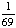None of the above. RESULTS BOX:
 2. Two cards are chosen at random from a deck of 52 cards without replacement. What is the probability that the first card is a jack and the second card is a ten?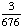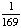None of the above. RESULTS BOX:
 3. On a math test, 5 out of 20 students got an A. If three students are chosen at random without replacement, what is the probability that all three got an A on the test?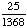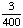None of the above. RESULTS BOX:
 4. Three cards are chosen at random from a deck of 52 cards without replacement. What is the probability of choosing an ace, a king, and a queen in order?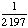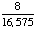None of the above. RESULTS BOX:
 5. A school survey found that 7 out of 30 students walk to school. If four students are selected at random without replacement, what is the probability that all four walk to school?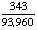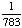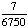None of the above. RESULTS BOX: